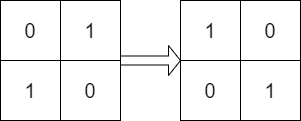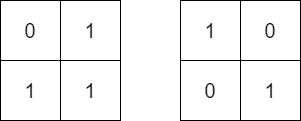# GeetCode Hub

Given two `n x n` binary matrices `mat` and `target`, return `true` if it is possible to make `mat` equal to `target` by rotating `mat` in 90-degree increments, or `false` otherwise.

Example 1:```Input: mat = [[0,1],[1,0]], target = [[1,0],[0,1]]
Output: true
Explanation: We can rotate mat 90 degrees clockwise to make mat equal target.
```

Example 2:```Input: mat = [[0,1],[1,1]], target = [[1,0],[0,1]]
Output: false
Explanation: It is impossible to make mat equal to target by rotating mat.
```

Example 3:```Input: mat = [[0,0,0],[0,1,0],[1,1,1]], target = [[1,1,1],[0,1,0],[0,0,0]]
Output: true
Explanation: We can rotate mat 90 degrees clockwise two times to make mat equal target.
```

Constraints:

• `n == mat.length == target.length`
• `n == mat[i].length == target[i].length`
• `1 <= n <= 10`
• `mat[i][j]` and `target[i][j]` are either `0` or `1`.

class Solution { public boolean findRotation(int[][] mat, int[][] target) { } }